# Numerical study of heat and mass transfer in underexpanded sonic free jet

Numerical study of heat and mass transfer in underexpanded sonic free jet

Omid Adibi Bijan Farhanieh Hossein Afshin

Center of Excellence in Energy Conversion, Sharif University of Technology, Iran

Corresponding Author Email:
Afshin@sharif.edu
Page:
959-968
|
DOI:
https://doi.org/ 10.18280/ijht.350432
|
Accepted:
|
Published:
31 December 2017
| Citation

OPEN ACCESS

Abstract:

In case of unexpected ruptures in high pressure tanks, choking phenomenon occurs in the cracked area which fluid velocity gets sonic and its pressure exceeds atmospheric pressure. Afterward, pressure of discharged gas quickly changes by crossing through compression and expansion shock waves. The main aim of this study was numerical simulation and investigation of this flow in a 3-step process: (a) Detailed analysis of numerical method, (b) Qualitative and quantitative analysis of velocity, pressure, temperature and turbulence intensity, in order to propose safety strategies and (c) parametric studies on the effects of tank pressure and nozzle geometry on flow structure. In numerical simulations, governing equations (conservation of mass, momentum, energy and equation of state) were discretized based on the finite volume method and flow variables (velocity, pressure, temperature and density) were calculated using density-based algorithm. Validation of numerical method was achieved by comparison with experimental data. The results showed that within 4.4 mm of cracked area, fluid temperature reached to its minimum value of 86 K which would freeze nearby equipment and would make them fragile. Also, parametric studies indicated that inlet pressure had direct relation with exhausted mass flow rate and its maximum occurred in nozzle angle of 3.82o.

Keywords:

numerical simulation, gas release, sonic free jets, high pressure tanks, shock waves

1. Introduction

Strict rules in design of fuel tanks and gas pipelines reduce number of accidents in these fuel transmission tools. Nevertheless, due to the amount of stored energy, smallest defect or carelessness in installation of equipment will lead to terrible disasters. For instance, in 2004, negligence of drilling workers caused a crack in Belgium methane pipelines. Thereafter, methane released to atmosphere and made major fire in the area which killed at least 15 people .

By perusing explosive events, it can be seen that after sabotage and terroristic acts, gas release from cracked tanks and pipelines is the main cause of these accidents. In other words, corrosions or cracks in tanks and pipelines lead to penetration of large amounts of gases to environment. With regards to potential energy of fuel and air mixture in environment, a spark or sudden temperature rise in the flammability areas will result severe explosions [2, 3].

Large number of casualties raised importance of the issue and many studies in the field of gas leakage from tanks and pipes have been performed. A preliminary study in this field was conducted by Crist et al. . They examined gas leakage from tanks with pressure up to 1000 bar. Results determined the position and diameter of Mach disks for different values of tank pressure. In literature, Mach disc refers to position of underexpanded sonic free jets where normal shock wave is formed. Eggins and Jackson  investigated expansion of sonic free jets in more details. They used the laser-Doppler velocimetry technique and measured discharge rate from high pressure tank (up to 6.6 bar). Yu et al.  used planar laser-induced fluorescence to visualize flow pattern of high pressure gas which was injected into an engine cylinder.

Moreover, various analytical studies have been conducted in the field of underexpanded sonic free jets. Joe and Ahn  developed a simple analytical model to explore leakage regimes from high pressure pipes. In this research, by combination of momentum and continuity equations as well as assuming one dimensional flow, the discharge rate was estimated. Yuhu et al.  presented three mathematical models to predict discharge rate for three different subsonic, sonic and supersonic cases. In subsequent studies [9, 10], by using real gas equation of states (EOSs), improved analytical models were developed which determined discharge rate and velocity field with more accuracy.

In past decade, with advancements in computational fluid dynamics (CFD) and developments in computer hardware, studies have shifted toward numerical simulation. In contrast to analytical studies, numerical methods can predict structure of flow with more realistic assumptions and with more details. One of the most important numerical studies in this field was accomplished by Wilkening and Baraldi . They simulated leakage of methane and hydrogen in 2D and 3D cases. Results indicated that due to higher flammability limits and internal energy of hydrogen, cracks in pipes transmitting hydrogen is more dangerous than those in methane pipes. Venetsanos et al.  examined different scenarios of gas release and dispersion. In this study, probability of fire creation due to emission of hydrogen and methane from passenger vehicles investigated in confined and unconfined spaces. Heitsch et al.  studied flammability regions of a research laboratory by numerical methods. They simulated leakage of hydrogen from high pressure pipes in a confined environment. Further numerical studies in the field of gas release and diffusion were performed by other researchers [13, 14]. It should be noted that in most of previous studies, for simplification of numerical solution, effects of shock waves on overall structure of sonic free jets were neglected. In fact, the gas velocity at the cracked area was assumed to be sonic and its pressure was assumed to be atmospheric.

The main aim of this study was numerical investigation of underexpanded sonic free jets at the exhaust region of high pressure tanks. In the simulations, effects of shock waves on jet expansion were considered. By simulation of shock waves and prediction of flow pattern in near field of cracked area, more accurate structure of exhausting gas would become clear. In the first part of the paper, numerical strategies in simulation of this flow were explored. In the second part, by comparing numerical results with experimental data, its accuracy was evaluated. Then, specification of flow near the cracked area was explored and some parametric studies were also performed.

2. Material and Methods

2.1 Problem description

In the present study, gas leakage from a high pressure gas reservoir was simulated according to the conditions of Eggins and Jackson’s  benchmark test (Figure 1). In this experiment, due to large dimensions of the tank versus crack diameter, pressure of reservoir was nearly constant (6.6 atm).

2.2 Governing equations

Due to the formation of shock waves in exhausted flow from high pressure tanks, compressibility of flow should be considered. To simulate compressible flow, in addition to continuity and momentum equations, energy equation is also must be solved . In Eq(1) to Eq(3) conservation of mass, momentum and energy for unsteady, viscous and compressible flows were presented, respectively :

$\frac{\partial \rho}{\partial t}+\frac{\partial}{\partial x_{i}}\left(\rho u_{i}\right)=0$             (1)

$\frac{\partial}{\partial t}\left(\rho u_{i}\right)+u_{i} \frac{\partial}{\partial x_{i}}\left(\rho u_{j}\right)=-\frac{\partial P}{\partial x_{i}}+\frac{\partial \tau_{i j}}{\partial x_{i}}+\rho g_{i}$              (2)

$\frac{\partial}{\partial t}(\rho e)+u_{i} \frac{\partial}{\partial x_{i}}(\rho e)=-\frac{\partial q_{i}}{\partial x_{i}}-P \frac{\partial u_{i}}{\partial x_{i}}-\tau_{i j} \frac{\partial u_{j}}{\partial x_{i}}$              (3)

In these equations $\rho, u_{i}, P, \tau_{i j}, e$ and $q_{i}$ are fluid density, velocity vector, pressure, shear tension tensor, internal energy and heat flux vector, respectively.

2.3 Fluid properties

Due to compressibility of sonic jets, fluid density must be added to unknown variables of the problem. To get a unique result from numerical simulations, an auxiliary equation like EOS should be solved with above mentioned governing equations. Ideal gas EOS is the simplest EOS for gases at high temperatures and low pressures. But, by pressure increment and temperature decrement, the accuracy of results gets far away from experimental data. To increase solution’s accuracy, several EOSs for real gas streams are provided. Families of cubic EOSs are one of the most practical EOSs which despite the simplicity can predict properties of fluids with acceptable accuracy .

In this study, in order to increase the accuracy of numerical solutions, Aungier-Redlich-Kwong (ARK) EOS was used. This equation is one of cubic EOSs that was developed by Aungier  for flows with sharp changes in pressure and temperature. In this equation, by adding coefficient c, weakness of former equations in prediction of fluid properties near the critical point was resolved. In Eq(4) to Eq(9) ARK EOS and its related coefficients were presented :

$P=\frac{\overline{\mathrm{R}} T}{V-b+c}-\frac{a(T)}{V(V+b)}$           (4)

$a(T)=a_{0} T_{\mathrm{r}}^{-m}$           (5)

$b=0.08664 \overline{\mathrm{R}} T_{\mathrm{c}} / P_{\mathrm{c}}$            (6)

$c=\frac{\overline{\mathrm{R}} T_{\mathrm{c}}}{P_{\mathrm{c}}+\frac{a_{0}}{V_{\mathrm{c}}\left(V_{\mathrm{c}}+b\right)}}+b-V_{\mathrm{c}}$           (7)

$a_{0}=0.42747 \overline{\mathrm{R}}^{2} T_{\mathrm{c}}^{2} / P_{\mathrm{c}}$             (8)

$m=0.4986+1.1735 \overline{\omega}+0.4754 \overline{\omega}^{2}$           (9)

In these equations T, V and $\overline{\mathrm{R}}$ are temperature, specific volume and universal gas constant, respectively. Also, c and r indexes represent critical point and reduced quantities of fluid, respectively. Moreover a, b and c are coefficients of ARK EOS. In Eq(9), $\overline{\omega}$ is eccentricity factor which was used to consider the asymmetric effects of gas molecules. To calculate this factor, Eq(10) was used :

$\overline{\omega}=-\log \left(P_{\mathrm{r}}^{\mathrm{st}}\right)-1$ at $T_{r}=0.7$            (10)

2.4 Turbulence modeling

Regard to mixing and entrainment phenomenon in free jets and also due to overcoming of inertia forces to viscous forces, gas leakage from tanks has turbulent nature . In numerical modeling using Boussinesq approximation, turbulence terms in momentum equations (Reynolds stresses) get related to gradient of average velocities according to Eq(11) [20, 21]:

$-\rho \overline{u_{i}^{\prime} u_{j}^{\prime}}=\mu_{t}\left(\frac{\partial \overline{u}_{i}}{\partial x_{j}}+\frac{\partial \overline{u}_{j}}{\partial x_{i}}\right)-\frac{2}{3}\left(\rho k+\mu_{t} \frac{\partial \overline{u}_{k}}{\partial x_{k}}\right) \delta_{i j}$              (11)

In this equation $u_{i}^{\prime}$ and $\overline{u}_{i}$are fluctuation and average values of velocity vector and $\mu_{t}$ is called turbulence viscosity. In the simulations, for calculation of turbulence viscosity Realisable k-epsilon and SST k-omega turbulence models were used. These models, despite the simplicity, can predict the structure of flow with good accuracy. Governing equation for k- epsilon model was presented in Eq(12) [21-23]:

$\mu_{t}=\rho C_{\mu} \frac{k^{2}}{\varepsilon}$            (12)

which k and $\varepsilon$ are turbulence kinetic energy and turbulence dissipation rate as well as could be calculated through Eq(13) and Eq(14) [21, 22]:

\begin{aligned} \frac{\partial}{\partial t}(\rho k) &+\frac{\partial}{\partial x_{j}}\left(\rho k \overline{u}_{j}\right) \\ &=\frac{\partial}{\partial x_{i}}\left[\left(\mu+\frac{\mu_{t}}{\sigma_{k}}\right) \frac{\partial k}{\partial x_{i}}\right]+G_{k}+G_{b}-\rho \varepsilon-Y_{M}+S_{k} \end{aligned}        (13)

\begin{aligned} \frac{\partial}{\partial t}(\rho \varepsilon) &+\frac{\partial}{\partial x_{j}}\left(\rho \varepsilon \overline{u}_{j}\right)=\frac{\partial}{\partial x_{j}}\left[\left(\mu+\frac{\mu_{t}}{\sigma_{\varepsilon}}\right) \frac{\partial \varepsilon}{\partial x_{j}}\right] \\ &+\rho C_{1} S \varepsilon-\rho C_{2} \frac{\varepsilon^{2}}{k+\sqrt{v \varepsilon}}+C_{1 \varepsilon} \frac{\varepsilon}{k} C_{3 \varepsilon} G_{b}+S_{\varepsilon} \end{aligned}            (14)

In these equations, Gk and Gb are production terms due to gradients of average velocity and buoyancy effects. In Realisable k-epsilon model by adding $Y_{M}=2 \rho \varepsilon M_{t}^{2}$ to Eq(13), effects of flow compressibility were included . Constant parameters in this model are presented in Table 1.

Another two equation turbulence models is k-omega Shear Stress Transport (SST) where turbulence viscosity is related to turbulence kinetic energy and turbulence specific dissipation rate. Governing equations for SST k-omega models were presented in Eq(15) to Eq(17) :

$\mu_{t}=\frac{\rho k}{\omega} \frac{1}{\max \left[\frac{1}{\alpha^{*}}, \frac{S F_{2}}{\alpha_{1} \omega}\right]}$             (15)

$\frac{\partial}{\partial t}(\rho k)+\frac{\partial}{\partial x_{i}}\left(\rho k \overline{u}_{i}\right)=\frac{\partial}{\partial x_{i}}\left(\Gamma_{k} \frac{\partial k}{\partial x_{i}}\right)+\tilde{G}_{k}-Y_{k}+S_{k}$            (16)

\begin{aligned} \frac{\partial}{\partial t}(\rho \omega) &+\frac{\partial}{\partial x_{i}}\left(\rho \omega \overline{u}_{i}\right) \\ &=\frac{\partial}{\partial x_{j}}\left(\Gamma_{\omega} \frac{\partial \omega}{\partial x_{j}}\right)+G_{\omega}-Y_{\omega}+D_{\omega}+S_{\omega} \end{aligned}             (17)

which $S_{k}$ and $S_{w}$ are source terms in the turbulence kinetic energy and turbulence specific dissipation rate equations, respectively. Using turbulence SST k-omega model is recommended for simulation of shear flows and flows which contain shock waves .

2.5 Discretization of equations

Governing equations of the problem was discretized by finite volume method where discretization methods and their accuracy for each term were presented in Table 2. Also, the under relaxation factors (URFs) were kept constant during the run.

2.6 Boundary conditions

Due to axial symmetry of geometry and boundary conditions, simulations are performed in the cylindrical coordinate system. In this case, there are no changes in angular parameters (∂/∂θ=0), therefore corresponding terms are ignored . By applying consistent boundary conditions to the system, geometry of the problem simplified as Figure 2. According to this figure, there were four types of boundary conditions which were explained in Table 3 and Table 4 .

2.7 Grid generation

In this study, structured method was used for grid generation. To avoid divergence of numerical results, aspect ratio of control volumes was set equal to one. Also, in order to predict flow more precisely, grids around the shock wave areas were generated with highest density. In other words, distance between grids gently was increased from cracked area to downstream of flow. In Figure 3 generated grids for the model were illustrated.

## 1.pngFigure 1. Schematics of the problem

## 2.png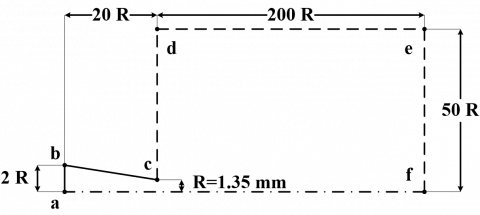Figure 2. Details of geometry in numerical model

## 3.jpg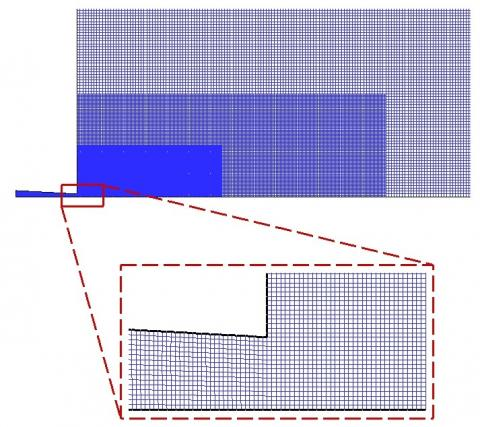Figure 3. General and magnified view of the grids

Table 1. Constants of realisable k-epsilon model 

 $C_{1 \varepsilon}$ $C_{2}$ $\sigma_{k}$ $\sigma_{\varepsilon}$ 1.4 1.9 1.0 1.2

Table 2. Discretization methods of governing equations

 Equations Discretization Methods Order of Accuracy Momentum MUSL Third Order Energy MUSL Third Order Turbulence kinetic energy Upwind Scheme Second Order Turbulence dissipation rate (k-ε model) Upwind Scheme Second Order Turbulence specific dissipation rate (k-ω model) Upwind Scheme Second Order

Table 3. Boundary conditions of the problem

 Name Type Description ab Constant Pressure Total pressure & temperature are equal to the reservoir conditions. bc Rigid Wall No-slip condition for velocity & adiabatic condition for temperature cdef Pressure Far-Field The boundary is taken to be far away from the nozzle outlet.. fa Axisymmetric Zero normal gradients is considered at this boundary

Table 4. Main parameters of the numerical model

 Parameter Symbol Value Pressure of reservoir (atm) P0 6.6 Pressure of ambient (atm) P∞ 1.0 Temperature of reservoir (K) T0 293 Initial temperature of ambient (K) T∞ 293

2.8 Time steps and grid sizes

The thickness of shock waves is in order of free molecular length and fluid properties sharply change by crossing through them . Therefore, to predict flow changes appropriately, grid sizes in the area near to the nozzle is intended equal to one micrometer. In Figure 3 small size of control volumes near the nozzle area was clearly demonstrated. To avoid divergence of numerical simulation, problem was solved as an unsteady case with time steps equal to one microsecond.

3. Results and Discussion

3.1 Thermodynamic properties

In this section predicted results of thermodynamic properties of gases by real gas and ideal gas EOSs were compared with experimental data of Lemmon et al. . In Figure 4 results of specific volume of air versus temperature are provided for pressures of 1, 10 and 100 atm. It is clear that at high pressures and low temperatures, difference between the results of ideal gas EOS and experiment are noticeable. Therefore, due to rapid changes in temperature and pressure in underexpanded sonic free jets, using ideal gas EOS is not suitable in current problem. Closer examination of Figure 4a and 4b showed that at T=81 and 105 K, sharp changes in specific volume of air were occurred which were due to fluid phase changes. The results indicated that the numerical method calculated the phase change points correctly and fluid properties in both liquid and gas phases were predicted in accordance with experimental data.

3.2 Grid independency

## 4.pngFigure 4. Specific volume of air versus temperature changes

To determine grid independency of numerical solution, simulations were achieved by 4 different types of grids. In Figure 5, axial velocity at distance of 2.7 mm from nozzle outlet was plotted versus radial distance from center line of jet stream. Nozzle outlet is end part of the nozzle which is connected to environment. This part was clearly shown in Figure 1. Figure 5 showed that by finning grid type 3 (with 103 thousand control volumes) results didn’t change significantly. Therefore, to reduce computational costs, future simulations were conducted with grid type 3.

3.3 Wall Yplus analysis

Due to high velocity gradients near walls, relatively fine grids must be generated in this area. To capture correct velocity profile wall Yplus should range from 30 to 300. In other words, wall adjacent cell should be located within the log-law layer . Wall Yplus is defined as Eq(18) :

$Y^{+}=\frac{u_{*} y}{v}$              (18)

which $u_{*}$, $V$ and y are friction velocity, kinematic viscosity and distance to nearest wall. In Figure 6 wall Yplus were plotted along nozzle wall for both Realisable k-epsilon and SST k-omega models. Results showed that wall Yplus value varies from 75 to 175 which higher values were related to areas with higher velocities (near to nozzle outlet). Based on numerical results it’s clear that mesh density near the wall were well adjusted.

3.4 Flow structure

In Figure 7 structure of flow at high pressure tanks outlet was shown. Figure 7a presents the result of experimental study of Eggins and Jackson  which was visualized by shadowgraphy technique. In this method, due to the changes in thermodynamic properties of fluids, flow pattern gets visible. In Figure 7b and 7c results of numerical simulations by Realisable k-epsilon and SST k-omega models were shown. These figures show the density contours of fluid which darker areas correspond to higher densities. By comparing numerical results with experimental data, it could be concluded that Realisable k-epsilon turbulence model predicted overall structure of flow with applicable approximation. In addition to acceptable prediction of flow structure, the SST k-omega turbulence model also predicted details of sonic free jets. The details include expansion fans, compression waves, Mach disk and slip lines which were predicted according to previous analytical studies .

## 5.png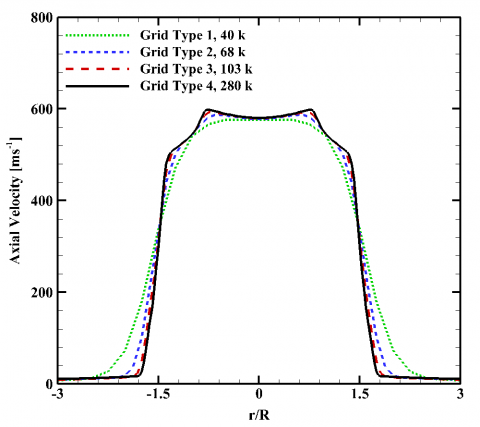Figure 5. Axial velocity versus distance from center line of jet stream

## 6.png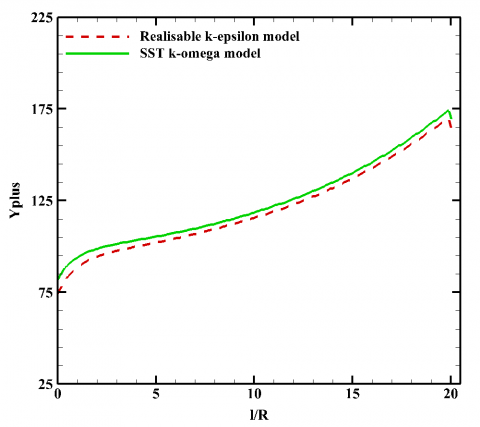Figure 6. Wall Yplus evolution along the nozzle length

3.5 Flow velocity field

Contours of velocity magnitude and path lines were presented in Figure 8. Results show that at exhaust region of the tank, flow was chocked and its velocity was got sonic (about 313 ms-1). Also, the fluid velocity before and after the exhaust region were respectively less and more than sound speed. This flow pattern can be easily explained according to choking phenomenon.

By comparing Figure 7 and Figure 8 it could be also observed that fluid velocity was severely reduced by passing through Mach disk area. The reason for this trend is combination of oblique shock waves and creation of normal shock wave at Mach disk area. In other words, normal shock waves always are followed by sharp velocity decrease.

For a closer look in velocity field, in Figure 9a and 9b velocity of fluid at upstream and downstream of Mach disk were plotted, respectively. These areas were indicated in Figure 8 by A-A and B-B lines. In Figure 9 the horizontal axis is the distance from center line of jet stream which position r/R=0 is consistent with the symmetry axis. By comparing numerical results with experimental data it could be seen that SST k-omega model predicted velocity profile better than Realisable k-epsilon model.

Moreover, scrutiny through Figure 8a showed that by getting away from the central axis of jet stream, fluid velocity gradually decreases. This process is similar to velocity profile of subsonic free jets . In contrast, Figure 9b shows that fluid velocity in middle region was reduced sharply. This area includes from the central axis of jet stream to slip line.

## 7.png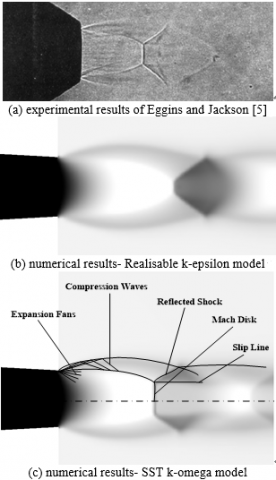Figure 7. Flow structure at outlet of high pressure tanks

## 8.png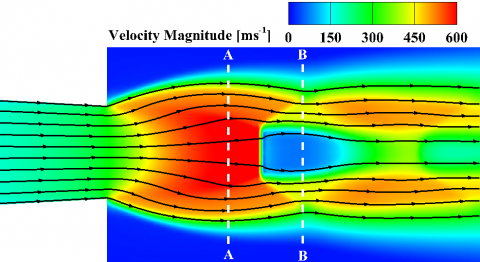Figure 8. Velocity magnitude countors and path lines

## 91.png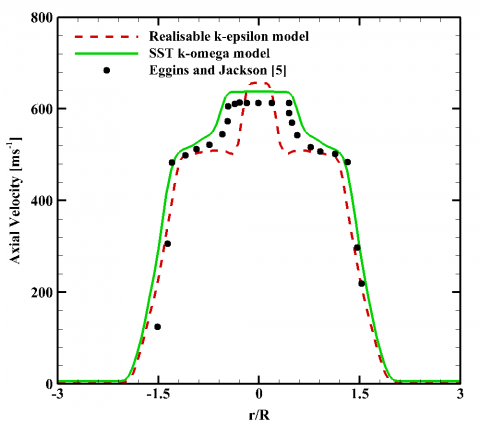(a) line A-A: upstream of Mach disk with 0.2 mm distance

## 92.png(b) line B-B: downstream of Mach disk with 0.2 mm distance

Figure 9. Axial velocity against distance from center line of jet stream

3.6 Flow pressure field

In the numerical simulations, in addition to velocity field other flow parameters such as pressure and temperature also could be achieved. Accordingly, static and total pressure contours at outlet of high pressure tanks were shown in Figure 10a and 10b, respectively. Results showed that fluid static pressure at nozzle outlet was higher than atmospheric pressure and it reduced in the downstream. This pressure reduction was due to expansion waves which fluid was passed through them. After collision of expansion waves with boundary of jet stream, they got reflected as compression waves. Combinations of these waves drove the Mach disk area. In this region, pressure of fluid increased extremely. Results also showed that flow with total pressure of 6.5 atm discharged to the atmosphere and after crossing Mach disk area, it suddenly dropped to 1.5 atm.

In Figure 11 static pressure at center of jet stream was plotted versus distance from nozzle outlet. It could be observed that due to compression and expansion waves, fluid pressure was oscillating in near field of nozzle outlet. By moving to downstream of the flow, due to shear forces, pressure fluctuations gradually reduced and finally its pressure got equal to ambient pressure.

## 101.png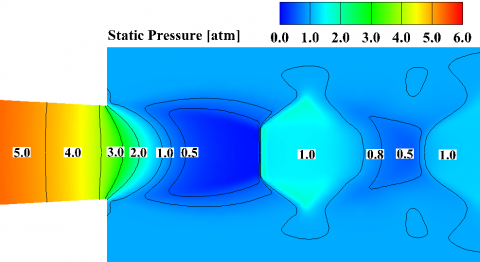(a) Static pressure

## 102.png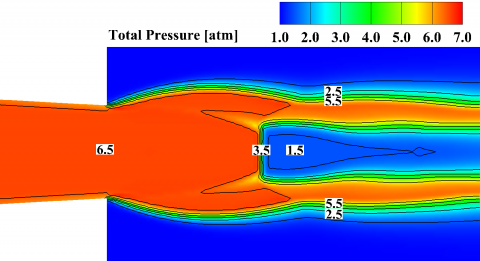(b) Total pressure

Figure 10. Pressure countors at outlet of high pressure tanks

## 11.pngFigure 11. Static pressure of jet steam versus distance from nozzle outlet

3.7 Flow temperature field

In Figure 12 static and total temperature contours were presented at the outlet of high pressure tanks. The results showed that in behindhand of the Mach disk, fluid temperature was reached to its minimum value. By comparing Figure 10a and Figure 12a, it could be seen that fluid pressure was also minimum in this area. In fact, pressure reduction was followed by temperature drop which this process could be explained by Joule-Thomson concept. Positive values of Joule-Thomson coefficient indicate fluids such as refrigerant which temperature of these fluids have direct relation with pressure Therefore, due to positive value of Joule-Thomson coefficient for air constituent (oxygen and nitrogen) , air temperature was reduced in expansion processes. Results also showed that in boundary layer region of jet stream, total temperature gradients were higher.

For a closer look at temperature distribution, in Figure 13 temperature changes at center of jet stream is plotted versus distance from the nozzle outlet. The results show that 4.4 mm from the outlet, fluid temperature is reduced to 86 K. Thus, in gas leakage from high pressure tanks and pipelines, freezing and fragility of equipment will happen in adjacent regions. Accordingly, in design of high pressure tanks and pipelines, insulation of sensitive equipment and installation of equipment with safe distance seems to be necessary.

## 121.png(a) Static temperature

## 122.png(b) Total temperature

Figure 12. Temperature countors at outlet of high pressure tanks

## 13.png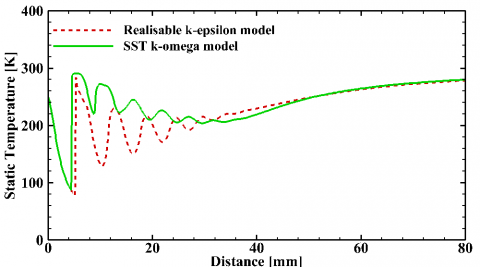Figure 13. Static temperature of jet steam versus distance from nozzle outlet

3.8 Turbulence field

TKE and TI countors at outlet of high pressure tanks are presented in Figure 14a and 14b, respectively. TKE is kinematic energy of velocity fluctuations and TI is turbulence intensity which was calculated using Eq(19):

$T I=\frac{u^{\prime}}{\overline{u}}$          (19)

In this equation $u^{\prime}$ and $\overline{u}$ are the root-mean-square of the turbulent velocity fluctuations and the mean velocity. Results show that due to high velocity fluctuations, TKE and TI are maximum at the boundaries of jet stream. In Figure 15 turbulence kinetic energy are plotted versus radial distance in different sections from nozzle outlet. It’s clear that the turbulence kinetic energiy increases by radious and reaches to its maximum at the boundary layers and then gradualy drops to ambient condition (TI=0). Also results indicate that by getting away from nozzle out, peak value of TKE reduces and its domain gets larger. In other words, by expansion of jet srtream in the atmosphere, maximim value of TKE reduces.

## 141.png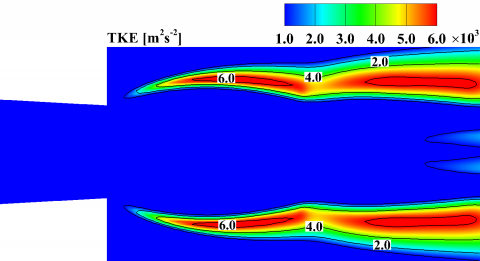(a) Turbulence kinetic energy

## 142.png(b) Turbulence intensity

Figure 14 Turbulence countors at outlet of high pressure tanks

## 15.pngFigure 15. Turbulence kinetic energy against distance from center line of jet stream

3.9 Parametric studies

To investigate the effects of inlet pressure on the flow structure some parametric simulations were performed. Therefore, beside the base condition (case B: Ptotal=6.6 atm), three different inlet pressures (case A: Ptotal=3.3 atm, case C: Ptotal=9.9 atm and case D: Ptotal=13.2 atm) were also considered in the simulations. Results of simulations were presented in Table 5. In this table, total and static pressure, total and static temperature, velocity magnitude, fluid density, TKE and mass flow rate were reported. Results showed that inlet pressure increases did not have any effects on fluid temperature and velocity because flow was chocked in nozzle outlet and fluid velocity was reached to its maximum value (sound speed). But pressure increases raised fluid density and as a result mass flow rate were also raised up. This process was clearly shown in Figure 16a. In this figure mass flow rate was plotted against different inlet pressures.

Table 5. Results of parametric study on inlet pressure variations

 Case Name Nozzle Inlet Nozzl Outlet Mass flow rate (gs-1) Pressure (atm) Pressure (atm) Temperature (K) Velocity (ms-1) Density (kgm-3) TKE (m2s-2) Total Static Total Static Total Static 3 3.3 3.27 3.26 1.73 293 244 313 2.50 84.86 4.49 B 6.6 6.54 6.53 3.46 293 244 313 4.99 74.39 8.91 C 9.9 9.81 9.82 5.19 293 244 313 7.48 68.79 13.38 D 13.2 13.07 13.11 6.92 293 244 314 9.98 65.15 17.86

For analyzing the effects of nozzle geometry on flow structure, beside the base condition (case B: L=20R), three different nozzle lengths (case E: L=15R, case F: L=10R and case G: L=5R) were also considered in the simulations. In these simulations other parameters such as nozzle inlet and outlet radius were kept constant. Therefore, by changing nozzle length, its angle was also changed. Results of these parametric simulations were presented in Table 6. Results indicated that geometry variation have negligible effects on flow parameters. But generally, the maximum release rate was occurred in case E (nozzle angle=3.82o) which was clearly demonstrated in Figure 16b. In this figure mass flow rate was plotted against different nozzle angles.

## 161.png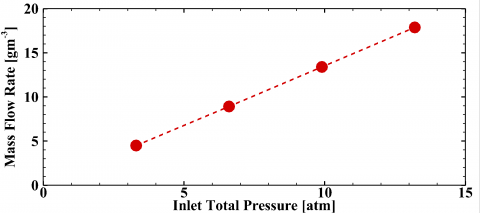(a) Inlet total pressure varation

## 162.png(b) Geometry (nozzle lengh and angle) variation

Figure 16. Mass flow rate diagram

Table 6. Results of parametric study on geometry variations

 Case Name Nozzle Geometry Nozzl Outlet Mass flow rate (gs-1) Lengh Angle (degree) Pressure (atm) Temperature (K) Velocity (ms-1) Density (kgm-3) TKE (m2s-2) Total Static Total Static B 20R 2.86 6.53 3.46 293 244 313 4.99 74.39 8.91 E 15R 3.82 6.56 3.55 293 246 308 5.09 69.93 8.93 F 10R 5.71 6.58 3.66 293 247 302 5.20 64.53 8.93 G 5R 11.32 6.59 3.83 293 250 291 5.38 52.49 8.85
4. Conclusions

In this study leakage process from high pressure tanks and pipelines were numerically investigated. The results showed that due to high pressure difference between reservoir and ambient, gas leaked by sonic velocity and higher pressure than atmosphere. Also, compression and expansion shock waves were observed in near field of cracked area. Further results were summarized in the following paragraphs:

• Existence of shock waves at outlet of high pressure tanks and pipelines make the structure of flow different from typical subsonic free jets. In this case, up to 40 mm from the cracked point, rapid changes in velocity, pressure and temperature occurred. Changes in fluid temperature and pressure led to changes in fluid properties such as density.
• In the leakage process from high pressure tanks, due to sharp changes in fluid properties, using ideal gas EOS was not suitable. The numerical results declared that by using Aungier-Redlich-Kwong EOS, fluid properties were estimated in accordance with experimental data.
• In sonic free jets, effects of flow compressibility should be considered in turbulence modeling. Numerical results| indicated that SST k-omega turbulence model could predict flow details more precisely than Realisable k-epsilon turbulence model does. The SST k-omega turbulence model predicted the position of Mach disk and flow structure according to experimental data.
• According to the countors of turbulence results, it was shown that maximum turbulence kinetic energy and turbulence intensity were occurred in boundary layer of free jet. Also, results indicated that maximum value of TKE decreased by getting away from nozzle outlet.
• Parametric studies showed that inlet pressure increase didn’t change fluid velocity and temperature. But due to density increment, mass flow rate had direct relation with inlet pressure.

Parametric simulations showed that nozzle geometry did not have any insignificant effects on release rate. However, release rate was maximum in case E with nozzle angle and length of 3.82o and 15R, respectively (R was radius of nozzle outlet).

Nomenclature
 a(T) temperature coefficient of ARK EOS, Pa mol2 kg-2 a0 constant of ARK EOS, Pa mol2 kg-2 b constant of ARK EOS, mol kg-1 c constant of ARK EOS, mol kg-1 C1, C2, C3, Cμ constants of turbulence equations D cross diffusion term in turbulence equations e internal energy, J m3 kg-1 F2 blending function in turbulence equations g gravity, m s-2 G, $\widetilde{G}$ generation of turbulence kinetic energy, kg m-1s-3 k turbulence kinetic energy, m2 s-2 L nozzle length, m m power constant of ARK EOS M Mach number P pressure, Pa q heat flux, W m-2 r radius, m R nozzle outlet radius, m $\overline{R}$ universal gas constant, J mol-1 K-1 S source term, W m-3 t time, s T temperature, K TI turbulence intensity, % TKE turbulence kinetic energy, m2 s-2 $u$ velocity, m s-1 $\overline{u}$ average velocity, m s-1 $u^{\prime}$ velocity fluctuation, m s-1 $u_{*}$ frictional velocity, m s-1 V specific volume, m3 mol-1 x position, m y distance from nearest wall, m Y dissipation term in turbulence equations, kg m-1 s-3 YM constant of turbulence equations, kg m-1 s-3 $Y^{+}$ wall Yplus Greek symbols $\alpha^{*}$ Reynolds number correction in turbulence equation $\alpha_{1}$ constants of turbulence equations $\Gamma$ effective diffusivity in turbulence equations $\delta$ Kronecker delta $\varepsilon$ turbulence dispersion rate, m2 s-2 $\theta$ angular coordinate μ dynamic viscosity, kg m-1s-1 v kinematic viscosity, m2 s-1 ρ density, kg m-3 $\sigma$ turbulence Prandtl numbers $\tau$ tension tensor, Pa $\omega$ turbulence specific dissipation rate, s-1 $\overline{\omega}$ eccentricity factor of ARK EOS Subscripts and superscripts 0 reservoir condition b buoyancy effect c critical point i coordinate index j coordinate index k coordinate index, turbulence kinetic energy index r reduced state of fluid sat saturated state of fluid t turbulence index total total condition of flow $\varepsilon$ turbulence dispersion rate index $\omega$ turbulence specific dissipation rate index $\infty$ ambient condition
References

 Wilkening H., Baraldi D. (2007). CFD modelling of accidental hydrogen release from pipelines, International Journal of Hydrogen Energy, Vol. 32, No. 13, pp. 2206-2215. DOI: 10.1016/j.ijhydene.2007.04.022

 Transportation Research Board (1988). Pipelines and Public Safety, The National Academies Press, Washington, DC, USA, Special Report 219.

 Organisation for Economic Co-operation and Development (1997). Report of the OECD workshop on pipelines (prevention of, preparedness for, and response to releases of hazardous substances), Paris, France, Series on Chemical Accidents No. 2.

 Crist S., Glass D.R., Sherman P.M. (1966). Study of the highly underexpanded sonic jet, AIAA Journal, Vol. 4, No. 1, pp. 68-71. DOI: 10.2514/3.3386

 Eggins P.L., Jackson D.A. (1974). Laser-doppler velocity measurements in an under-expanded free jet, Journal of Physics D: Applied Physics, Vol. 7, No. 14, pp. 1894-1906. DOI: 10.1088/0022-3727/7/14/308

 Yu J., Vuorinen V., Kaario O., Sarjovaara T., Larmi M. (2013). Visualization and analysis of the characteristics of transitional underexpanded jets, International Journal of Heat and Fluid Flow, Vol. 44, No.  pp. 140-154. DOI: 10.1016/j.ijheatfluidflow.2013.05.015

 Jo Y.D., Ahn B.J. (2003). A simple model for the release rate of hazardous gas from a hole on high-pressure pipelines, Journal of Hazardous Materials, Vol. 97, No. 1, pp. 31-46. DOI: 10.1016/S0304-3894(02)00261-3

 Yuhu D., Huilin G., Jing’en Z., Yaorong F. (2003). Mathematical modeling of gas release through holes in pipelines, Chemical Engineering Journal, Vol. 92, No. 1, pp. 237-241. DOI: 10.1016/S1385-8947(02)00259-0

 Mahood H.B., Rasheed F.L., Abbas A.K. (2011). Analytical and numerical investigation of transient gas blow down, Modern Applied Science, Vol. 5, No. 5, pp. 64-72. DOI: 10.5539/mas.v5n5p64

 Sklavounos S., Rigas F. (2006). Estimation of safety distances in the vicinity of fuel gas pipelines, Journal of Loss Prevention in the Process Industries, Vol. 19, No. 1, pp. 24-31. DOI: 10.1016/j.jlp.2005.05.002

 Venetsanos A.G., Papanikolaou E., Delichatsios M., Garcia J., Hansen O., Heitsch M., Huser A., Jahn W., Jordan T., Lacome J.M. (2009). An inter-comparison exercise on the capabilities of CFD models to predict the short and long term distribution and mixing of hydrogen in a garage, International Journal of Hydrogen Energy, Vol. 34, No. 14, pp. 5912-5923. DOI: 10.1016/j.ijhydene.2009.01.055

 Heitsch M., Baraldi D., Moretto P. (2010). Numerical analysis of accidental hydrogen release in a laboratory, International Journal of Hydrogen Energy, Vol. 35, No. 9, pp. 4409-4419. DOI: 10.1016/j.ijhydene.2010.01.044

 Choi J., Hur N., Kang S., Lee E.D., Lee K.B. (2013). A CFD simulation of hydrogen dispersion for the hydrogen leakage from a fuel cell vehicle in an underground parking garage, International Journal of Hydrogen Energy, Vol. 38, No. 19, pp. 8084-8091. DOI: 10.1016/j.ijhydene.2013.02.018

 Visser D.C., Houkema M., Siccama N.B., Komen E.M.J. (2012). Validation of a FLUENT CFD model for hydrogen distribution in a containment, Nuclear Engineering and Design, Vol. 245, No.  pp. 161-171. DOI: 10.1016/j.nucengdes.2012.01.025

 Kuzmin A. (2016). Shock wave bifurcation in channels with a bend, Archive of Applied Mechanics, Vol. 86, No. 5, pp. 787-795. DOI: 10.1007/s00419-015-1062-z

 Wendt J. (2008). Computational Fluid Dynamics: An Introduction, Springer-Verlag, Berlin Heidelberg. DOI: 10.1007/978-3-540-85056-4

 Cengel Y.A., Boles M.A. (2015). Thermodynamics: An Engineering Approach, McGraw-Hill, New York.

 Aungier R.H. (1995). A fast, accurate real gas equation of state for fluid dynamic analysis applications, Journal of Fluids Engineering, Vol. 117, No. 2, pp. 277-281. DOI: 10.1115/1.2817141

 Faghani E., Maddahian R., Faghani P., Farhanieh B. (2010). Numerical investigation of turbulent free jet flows issuing from rectangular nozzles: The influence of small aspect ratio, Archive of Applied Mechanics, Vol. 80, No. 7, pp. 727-745. DOI: 10.1007/s00419-009-0340-z

 Hinze J. (1972). Turbulence, McGraw-Hill, New York.

 Launder B.E., Spalding D.B. (1974). The numerical computation of turbulent flows, Computer Methods in Applied Mechanics and Engineering, Vol. 3, No. 2, pp. 269-289. DOI: 10.1016/0045-7825(74)90029-2

 Launder B.E., Reece G.J., Rodi W. (1975). Progress in the development of a Reynolds-stress turbulence closure, Journal of Fluid Mechanics, Vol. 68, No. 03, pp. 537-566. DOI: 10.1017/S0022112075001814

 Liu L., Sun Z., Wan C., Wu J. (2016). Jet flow field calculation & mechanism analysis on hot-air drying oven based on rng k-ε model, International Journal of Heat and Technology, Vol. 34, No. 2, p. 9. DOI: 10.18280/ijht.330110

 Shih T.H., Liou W.W., Shabbir A., Yang Z., Zhu J. (1994), A new k-epsilon eddy viscosity model for high Reynolds number turbulent flows: Model development and validation, Cleveland, OH, USA, Document ID: 19950005029.

 Menter F.R. (1994). Two-equation eddy-viscosity turbulence models for engineering applications, AIAA Journal, Vol. 32, No. 8, pp. 1598-1605. DOI: 10.2514/3.12149

 Faghani E., Saemi S.D., Maddahian R., Farhanieh B. (2011). On the effect of inflow conditions in simulation of a turbulent round jet, Archive of Applied Mechanics, Vol. 81, No. 10, pp. 1439-1453. DOI: 10.1007/s00419-010-0494-8

 Kaliakatsos D., Cucumo M., Ferraro V., Mele M., Galloro A., Accorinti F. (2016). CFD analysis of a pipe equipped with twisted tape, International Journal of Heat and Technology, Vol. 34, No. 2, pp. 172-180. DOI: 10.18280/ijht.340202

 Lemmon E.W., Jacobsen R.T., Penoncello S.G., Friend D.G. (2000). Thermodynamic properties of air and mixtures of nitrogen, argon, and oxygen from 60 to 2000 K at pressures to 2000 MPa, Journal of Physical and Chemical Reference Data, Vol. 29, No. 3, pp. 331-385. DOI: 10.1063/1.1285884

 Zheng X., Jian X., Wei J., Wenzheng D. (2016). Numerical and experimental investigation of near-field mixing in parallel dual round jets, International Journal of Aerospace Engineering, Vol. 2016, No. DOI: 10.1155/2016/7935101

 Lee J., Lu T., Sun H., Miao G. (2011). A novel formula to describe the velocity profile of free jet flow, Archive of Applied Mechanics, Vol. 81, No. 3, pp. 397-402. DOI: 10.1007/s00419-010-0494-8